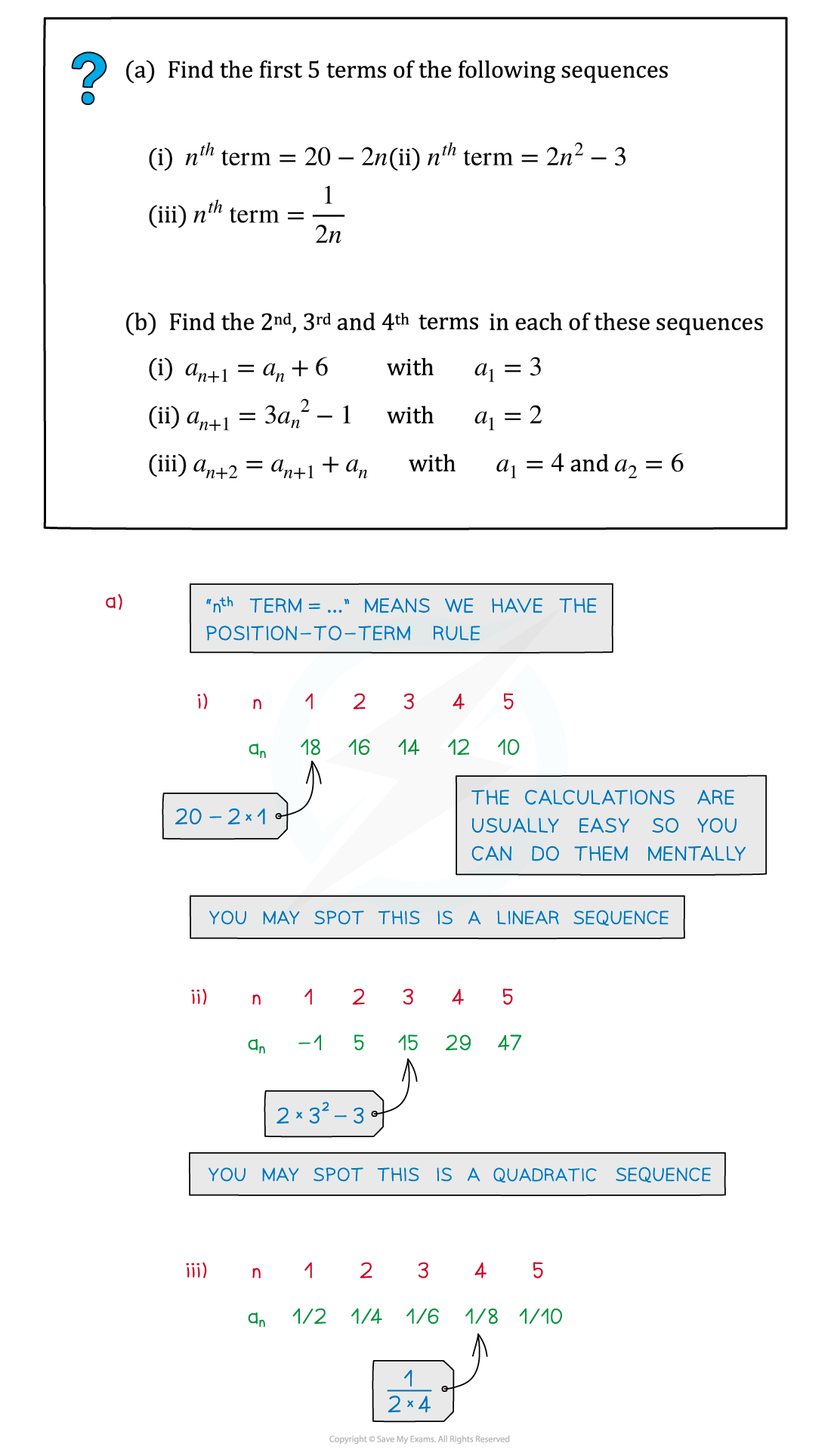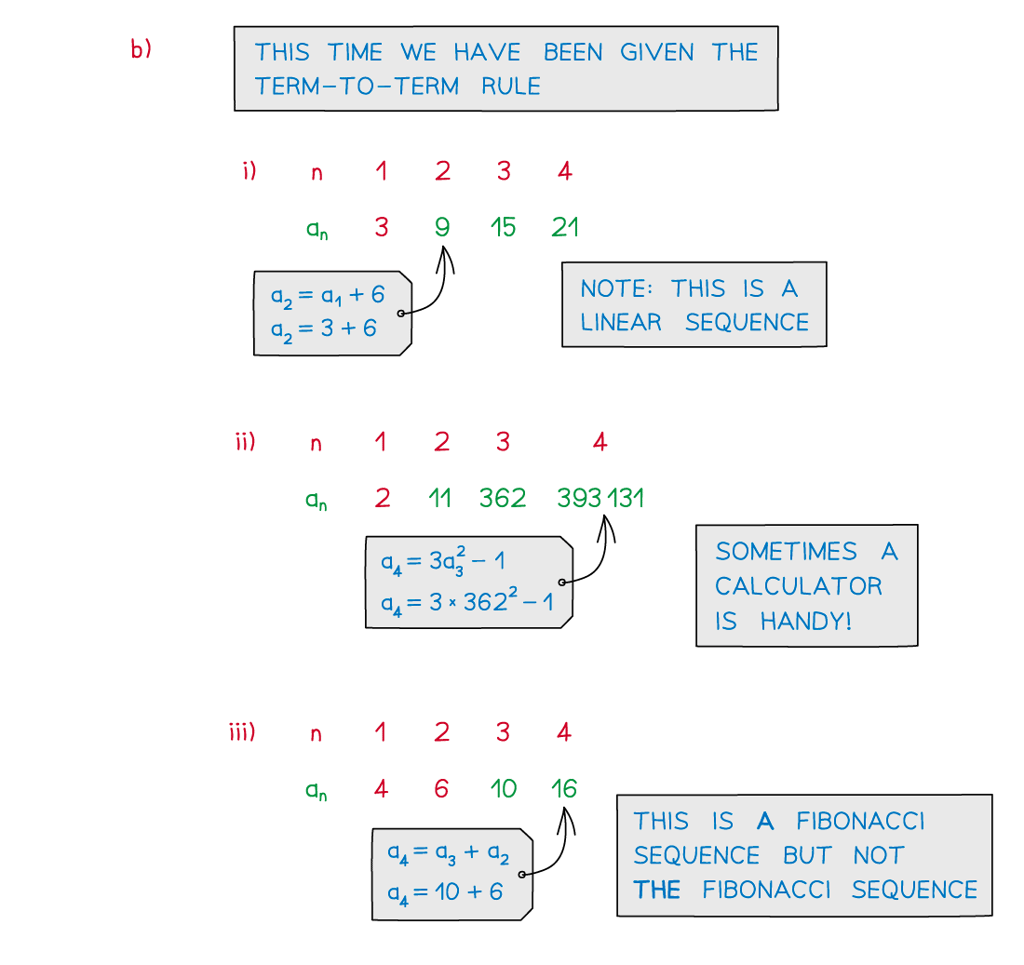# Edexcel IGCSE Maths 复习笔记 3.1.1 Sequences - Basics

Edexcel IGCSE Maths 复习笔记 3.1.1 Sequences - Basics

#### What are sequences?

• A sequence is an order set of (usually) numbers
• Each number in a sequence is called a term
• The location of a term within a sequence is called its position
• The letter n is often used for (an unknown) position

• Subscript notation is used to talk about a particular term
• a1 would be the first term in a sequence
• a7 would be the seventh term
• an would be the nth term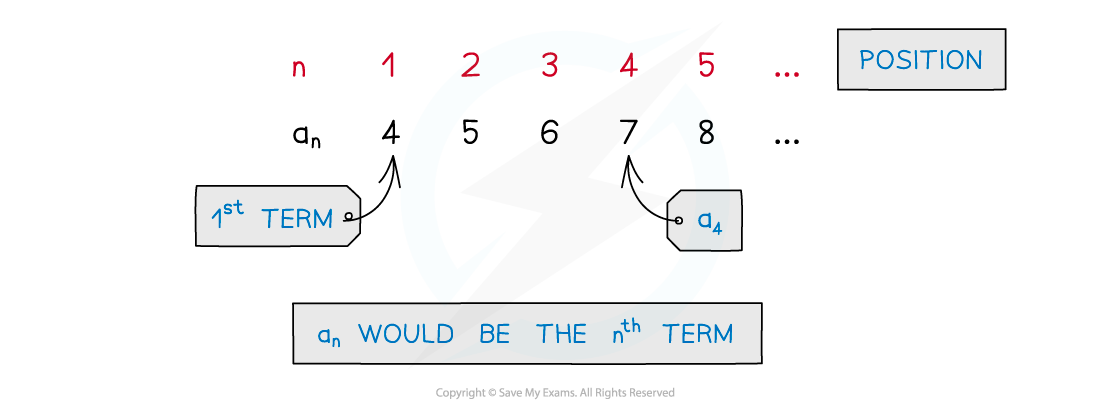#### What is a position-to-term rule?

• position-to-term rule gives the nth term of a sequence in terms of n
• This is a very powerful piece of mathematics
• With a position-to-term rule the 100th term of a sequence can be found without having to know or work out the first 99 terms!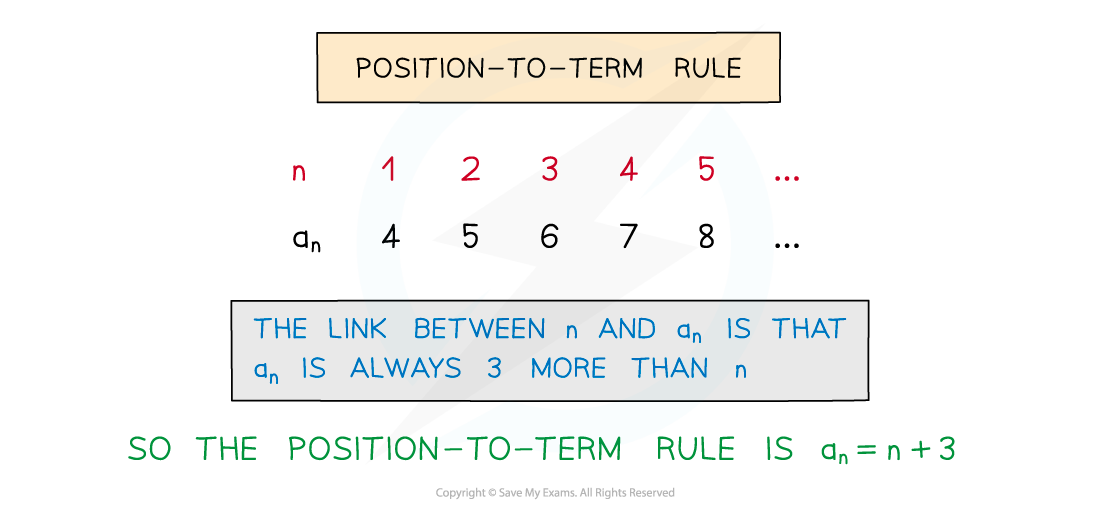#### What is a term-to-term rule?

• term-to-term rule gives the (n+1)th term in terms of the nth term
• ie an+1 is given in terms of an
• If a term is known, the next one can be worked out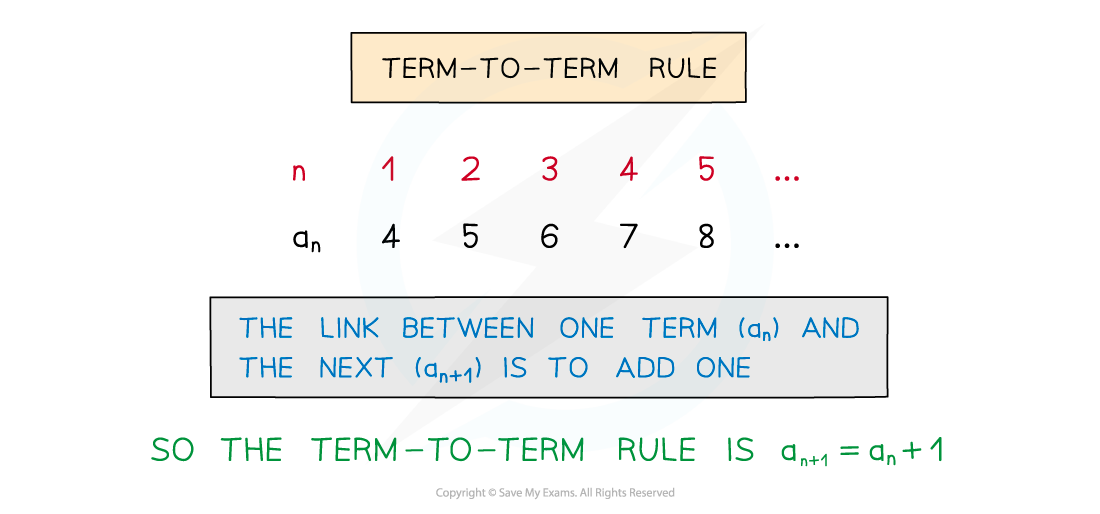#### How do I use the position-to-term and term-to-term rules?

• These can be used to generate a sequence
• From a given sequence the rules can be deduced
• Recognising and being aware of the types of sequences helps
• Geometric sequences
• Fibonacci sequences
• Other sequences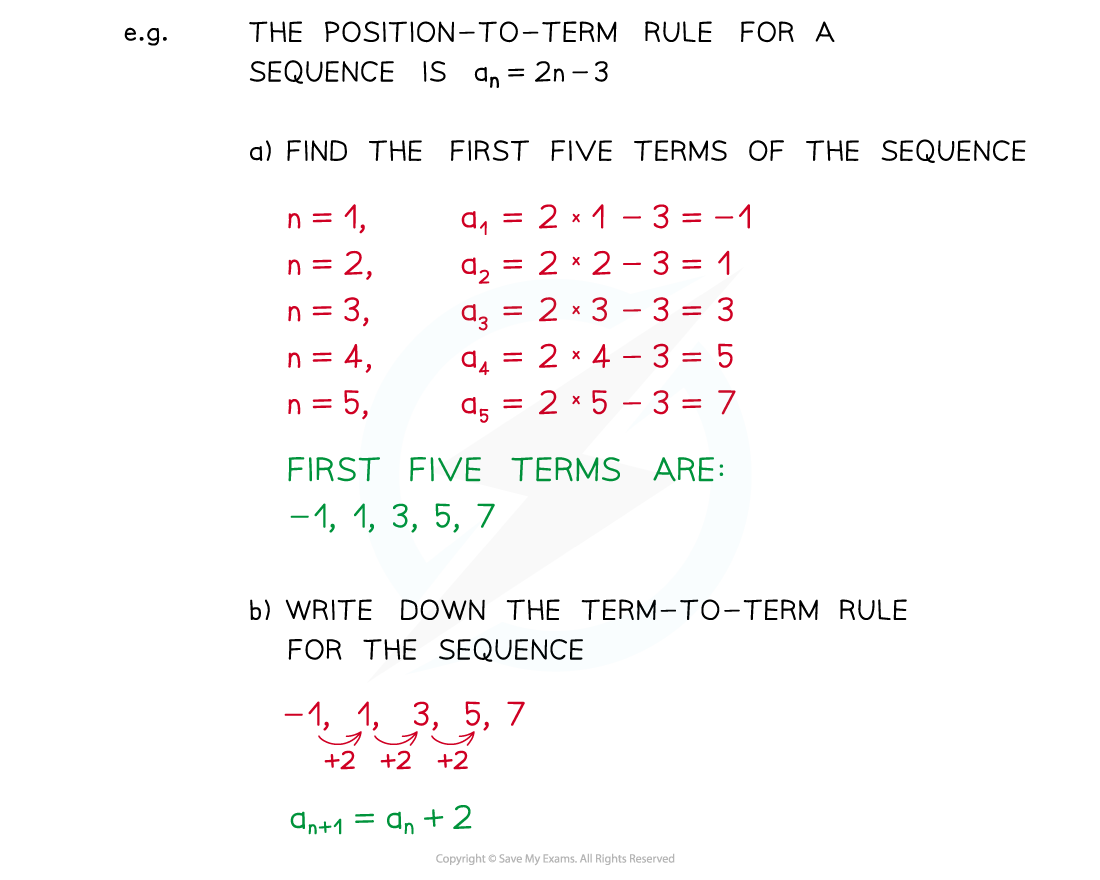#### Exam Tip

Write the position numbers above (or below) each term in a sequence.This will make it much easier to recognise and spot common types of sequence.